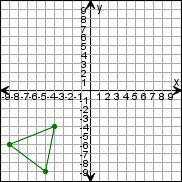Create a new printableGeometry
Math Worksheets

Sample - Click above to make a new math worksheet (PDF).
 Name _____________________________Date ___________________
Transformations
Draw the triangle after the transformations.

 1 Reflect the triangle over the x-axis.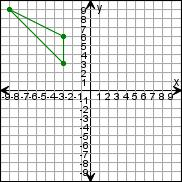2 Reflect the triangle over the x-axis.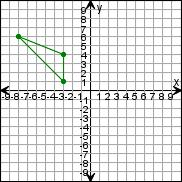3 Reflect the triangle over the y-axis.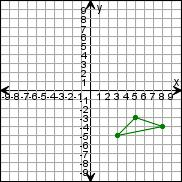4 * This is a pre-made sheet.Use the link at the top of the page for a printable page.
 5 Reflect the triangle over the y-axis.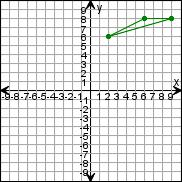6 Reflect the triangle over the x-axis.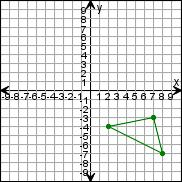7 Reflect the triangle over the x-axis.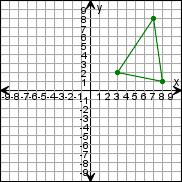8 Reflect the triangle over the y-axis.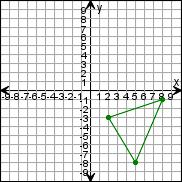9 Reflect the triangle over the x-axis.